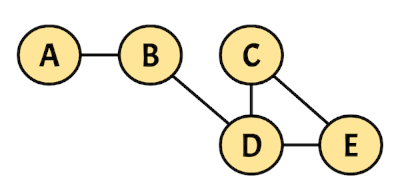# Lab #6: Graph Cycle Detection

### Objective

During this activity, students will be able to:

• Design and implement an algorithm that detects if an undirected graph contains a cycle.

## Description

This activity must be developed in the pre-assigned teams of two.

In a file called graph_cycle.py, write a Python function called has_cycle that takes as input an initial vertex and a undirected connected graph. The function traverses the graph in DFS (Depth-First Search) order and when it detects a cycle it returns a list with the vertices that conform the path of the cycle. If there are multiple cycles in the graph, the function returns the first one found. The function returns None if the graph contains no cycles.

For example, the following graph contains a cycle:If the DSF traversal starts at vertex A, the detected cycle has the following path:

DCED

Bear in mind that:

• A cycle can only occur if there are three or more vertices involved.

• The cycle’s path starts and ends with the same vertex.

The has_cycle function should be called like this:

has_cycle('A', { 'A': ['B'],
'B': ['A', 'D'],
'C': ['D', 'E'],
'D': ['B', 'C', 'E'],
'E': ['C', 'D']
})


Note that strings are used to represent the vertices, while the graph is represented as a dictionary where each key is a string and its associated value is a list of strings (the neighbours of the corresponding vertex).

The previous function call returns the following result:

['D', 'C', 'E', 'D']


Use the following code as a starting point:

# File: graph_cycle.py

from typing import Optional

Graph = dict[str, list[str]]

def has_cycle(initial: str, graph: Graph) -> Optional[list[str]]:
# The function's code goes here
...


Test your code using the tests in the file test_graph_cycle.py. Finally, make sure no type or PEP 8 style errors are produced by using the mypy and pycodestyle linters. You can run them at the terminal like this:

mypy cryptarithmetic_puzzle.py
pycodestyle cryptarithmetic_puzzle.py

## Deliverables

Place in a comment at the top of the graph_cycle.py source file the authors’ personal information (student ID and name), for example:

#----------------------------------------------------------
# Lab #6: Graph Cycle Detection
#
# Date: 03-Nov-2023
# Authors:
#           A01770771 Kamala Khan
#           A01777771 Carol Danvers
#----------------------------------------------------------


To deliver the graph_cycle.py file, please provide the following information: